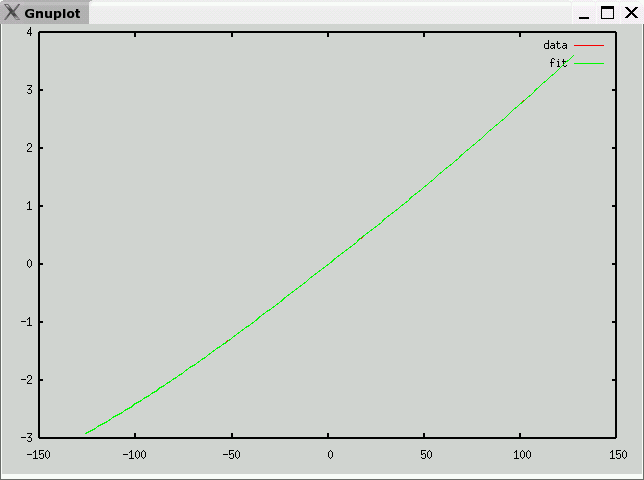# Type N Thermocouple Data and Polynomial

• Description
Ni-Cr-Si / Ni-Si-Mg
• Data source
National Institute of Science and Technology (NIST)
• Raw Data
• Octave format
• Curve fit
• mV -> temperature (C)
e is maximum error (in degrees C)
v1 andf v2 partition the domain into three segments, each with a polynomial fit
c is vector of coefficients: a10, a9, ... a0
Note: Each column of c corresponds to one range (<v1,v1 to v2, >v2)

`e = 1.1191v1 = -0.26000v2 = 7.6310c =   -3.8420e-01    1.7932e-06   1.5013e-13   -8.5101e+00   -7.0652e-05   -3.6475e-11   -8.1238e+01    1.1941e-03   3.7831e-09   -4.3750e+02   -1.1312e-02   -2.1762e-07   -1.4616e+03    6.5907e-02   7.4995e-06   -3.1382e+03   -2.4408e-01   -1.5180e-04   -4.3415e+03    5.7431e-01   1.3706e-03   -3.7755e+03   -7.9333e-01   1.4498e-02   -1.9495e+03   -3.6240e-01   -7.0781e-01   -4.9321e+02    3.8396e+01   3.6612e+01   -5.7426e+01    1.3776e-02   4.4746e+00 `• temperature -> mV (range -127C-127C)
e is maximum error (in mV)
c is vector of coefficients: a10, a9, ... a0

`e = 0.0010752c =   -1.6747e-22   -5.7538e-21    7.8795e-18    2.6677e-16   -1.4423e-13   -4.9429e-12    1.5144e-09    1.0610e-08    1.1520e-05    2.6083e-02   -9.2802e-04 `Previous page: Type K
Next page: Type R